# An alternative proof of a Tauberian theorem for the weighted mean summability of integrals over R_{+}

### Full PDFcreative_2020_29_1_45_50

Let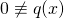be a nondecreasing function
on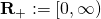such that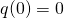and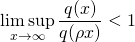for every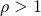.
Given a real- or complex- valued function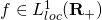, we define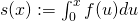and its weighted mean as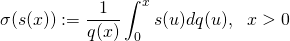provided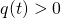.

If the limit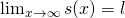exists, then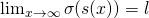also exists. However, the converse is not true in general.
The converse implication might hold under some appropriate conditions. These conditions are called Tauberian conditions
and theorems involving these conditions are called Tauberian theorems.

In this paper, we have given an alternative proof of the Tauberian theorem stating that
the existence offollows from that ofand a Tauberian condition.

If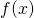is a real or complex-valued function, then this
Tauberian condition is one-sided or two-sided, respectively.